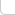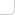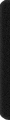# 某島

… : "…アッカリ～ン . .. . " .. .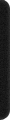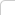August 18, 1453

## 【專題】Polya 計數定理

### References:

。。統計在某個置換群作用下。。計算不等價的著色方案數的問題。。。
。。可以通過 Burnside 定理。。轉化為枚舉每一個置換。。。統計在該置換作用下等價的著色方案數的問題。。
。。而 Polya 定理則是巧妙的利用同一循環內著色必須相同這個事實，進一步簡化後者的計算。。。

——————

## Burnside 定理：

$$! |G| N(G, C) = \sum_{f\in g}|C(f)|$$

## Polya 定理：

$$! |C(f)| = k^r$$。。。

——————

## 證明：

##### 引理 1：

$$gc = fc$$ 當且僅當 $$f^{-1} * g$$ 屬於 $$G(c)$$。

##### 推論 2：

$$! N(c) = |{f*c: f\in G}| = \frac{|G|}{|G(c)|}$$。

##### Burnside 定理：

。。考察。。所有滿足 $$f*c = c$$ 的二元組 $$(f, c)$$。。雙計數。。

$$! |G(c)| N(c) = |G|$$
$$!\sum_{c\in C} \frac{1}{N(c)} = N(G,C)$$ （因為每個非等價著色對左式的貢獻為 1。）

inline LL f(DB x){
return (pow(x, 6) + 3 * pow(x, 4) + 12 * pow(x, 3) + 8 * pow(x, 2)) / 24;
}

int main() {
int n; while (cin >> n && n){
cout << f(n) << endl;
}
}



HOJ 2647. Megaminx

inline LL f(DB x){
return (pow(x, 12) + 15 * pow(x, 6) + 44 * pow(x, 4)) / 60;
}

int main() {
int n; while (cin >> n){
cout << f(n) << endl;
}
}


SGU 294.

SGU 208. Toral Tickets
http://acm.hust.edu.cn:8080/judge/problem/viewProblem.action?id=14886
http://acm.hust.edu.cn:8080/judge/problem/viewSource.action?id=631448